# Getting Started with NNS: Correlation and Dependence

library(NNS)
library(data.table)
require(knitr)
require(rgl)
require(meboot)
require(tdigest)
require(dtw)

# Correlation and Dependence

The limitations of linear correlation are well known. Often one uses correlation, when dependence is the intended measure for defining the relationship between variables. NNS dependence NNS.dep is a signal:noise measure robust to nonlinear signals.

Below are some examples comparing NNS correlation NNS.cor and NNS.dep with the standard Pearson’s correlation coefficient cor.

## Linear Equivalence

Note the fact that all observations occupy the co-partial moment quadrants.

x = seq(0, 3, .01) ; y = 2 * x

cor(x, y)
##  1
NNS.dep(x, y, print.map = TRUE)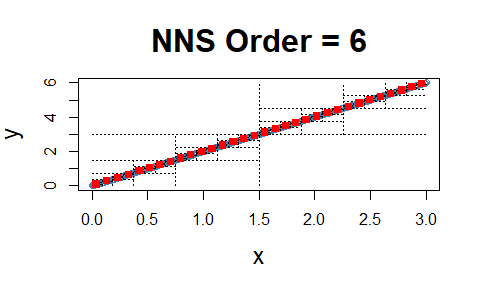## $Correlation ##  1 ## ##$Dependence
##  1

## Nonlinear Relationship

Note the fact that all observations occupy the co-partial moment quadrants.

x=seq(0, 3, .01) ; y = x ^ 10

cor(x, y)
##  0.6610183
NNS.dep(x, y, print.map = TRUE)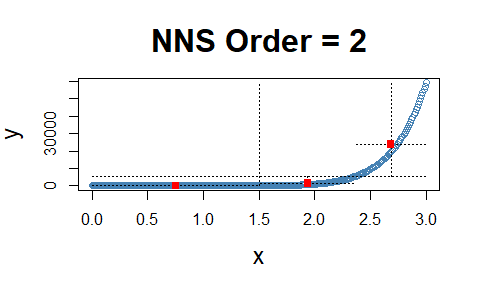## $Correlation ##  0.9458022 ## ##$Dependence
##  0.9458022

## Dependence

Note the fact that all observations occupy only co- or divergent partial moment quadrants for a given subquadrant.

set.seed(123)
df <- data.frame(x = runif(10000, -1, 1), y = runif(10000, -1, 1))
df <- subset(df, (x ^ 2 + y ^ 2 <= 1 & x ^ 2 + y ^ 2 >= 0.95))
NNS.dep(df$x, df$y, print.map = TRUE)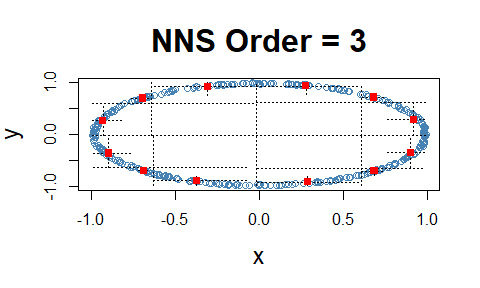## $Correlation ##  0.08959287 ## ##$Dependence
##  0.912399

# Multi-Dimensional Dependence

These partial moment insights permit us to extend the analysis to multivariate instances. This level of analysis is simply impossible with Pearson or other rank based correlation methods, which are restricted to pairwise cases.

set.seed(123)
x <- rnorm(1000); y <- rnorm(1000); z <- rnorm(1000)
NNS.dep.hd(cbind(x, y, z), plot = TRUE, independence.overlay = TRUE)
## $actual.observations ##  262 ## ##$independent.null
##  250
##
## $Dependence ##  0.016 # p-values for NNS.dep() p-values and confidence intervals can be obtained from sampling random permutations of $$y \rightarrow y_p$$ and running NNS.dep(x,$$y_p$$) to compare against a null hypothesis of 0 correlation, or independence between $$(x, y)$$. ## p-values for [NNS.dep] x <- seq(-5, 5, .1); y <- x^2 + rnorm(length(x)) nns_cor_dep <- NNS.dep(x,abs (y), print.map = TRUE)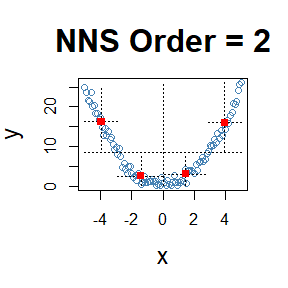nns_cor_dep ##$Correlation
##  0.007618383
##
## $Dependence ##  0.4972606 ## Create permutations of y y_p <- replicate(100, sample.int(length(y))) ## Generate new correlation and dependence measures on each new permutation of y nns.mc <- apply(y_p, 2, function(g) NNS.dep(x, y[g])) ## Store results cors <- unlist(lapply(nns.mc, "[[", 1)) deps <- unlist(lapply(nns.mc, "[[", 2)) ## View results hist(cors) abline(v = LPM.VaR(.025,0, cors), col = 'red') abline(v = UPM.VaR(.025,0, cors), col = 'red')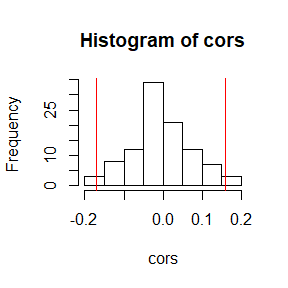## Left tailed correlation p-value cor_p_value <- LPM(0, nns_cor_dep$Correlation, cors)
cor_p_value
##  0.58
## Right tailed correlation p-value
cor_p_value <- UPM(0, nns_cor_dep$Correlation, cors) cor_p_value ##  0.42 ## Confidence Intervals ## For 95th percentile VaR (both-tails) see [LPM.VaR] and [UPM.VaR] ## Lower CI LPM.VaR(.025, 0, cors) ##  -0.1701219 ## Upper CI UPM.VaR(.025, 0, cors) ##  0.1594297 hist(deps) abline(v = LPM.VaR(.025,0, deps), col = 'red') abline(v = UPM.VaR(.025,0, deps), col = 'red')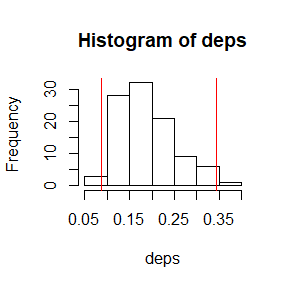## Left tailed dependence p-value dep_p_value <- LPM(0, nns_cor_dep$Dependence, deps)
dep_p_value
##  1
## Right tailed dependence p-value
dep_p_value <- UPM(0, nns_cor_dep\$Dependence, deps)
dep_p_value
##  0
## Confidence Intervals
## For 95th percentile VaR (both-tails) see [LPM.VaR] and [UPM.VaR]
## Lower CI
LPM.VaR(.025, 0, deps)
##  0.08597743
## Upper CI
UPM.VaR(.025, 0, deps)
##  0.3432286